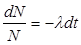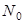# Deduce the law of radioactive disintegration.## The Law of Radioactivity Disintegration:

Let, N be the number of atoms present in the radio element at a given instant t. Then the rate of decrease,

Here, λ is the disintegration constant. It is defined as the ratio of the amount of the substance which disintegrates in a unit time to the amount of substance present.

The equation (i) can be written as,Integrating,

Let, there arenumber of atoms present initially.

Then, when t=0, N=N0

Substituting the value of C in equation (ii),Thus the number of atoms of a given radioactive substance decreases exponentially with time.